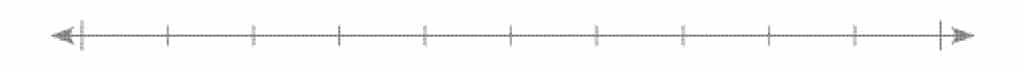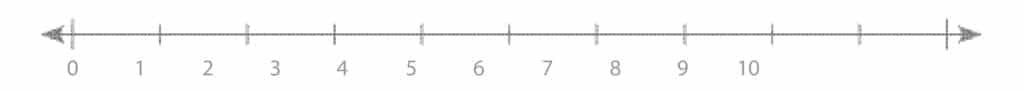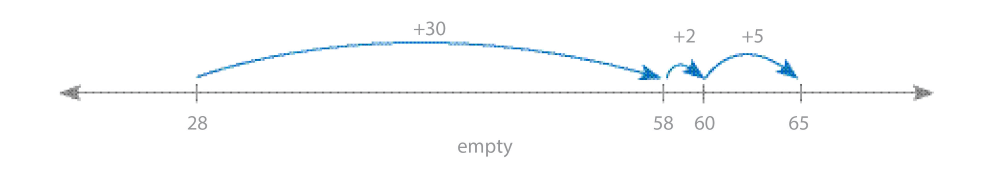# Number Lines

#### • Number: Counting, Operations, Addition, Subtraction, Multiplication, Division, Fractions, Equivalence

Typically, children begin working with number tracks and move on to number lines. A number track is associated with counting as typically students count moves along the track. Number lines allow students to make use of fraction and decimal fraction thinking, that is, you can show numbers like 1 3/4 and 3.5.

### Mathematical Language

Addition, subtraction, multiplication (‘lots of’), division, counting 1, 2, 3, etc, multiple, pattern, count on, count back.

### Using Number Lines

Number Lines vary from “Empty” or unstructured lines, where students place their own calibration and numbers on them:

• calibrated, but unnumbered lines• structured (calibrated and numbered) linesEach type of number line has a different purpose. For example a student may use an Empty number line to show the process of adding 28 + 37.### Typical Classroom Requirements

#### A class set:

– one number line 0-20 per pair of students
– one number line 0-120 per group of 4 students